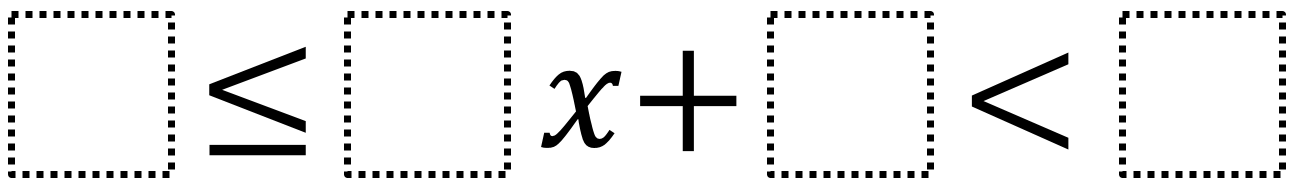# Compound Inequalities 2

Directions: Using the digits 1 to 9, at most one time each, make two compound inequalities that are equivalent to 2 ≤ x < 4.### Hint

How does changing the coefficient affect the resulting value?
How does changing the constant next to x affect the resulting value?

4 ≤ 1x + 2< 6 and 5 ≤ 2x + 1< 9.

Source: Robert Kaplinsky

## Difference of Squares and Sum of Cubes

Directions: Using the digits 0 to 9 at most one time each, place a digit …

1.2.